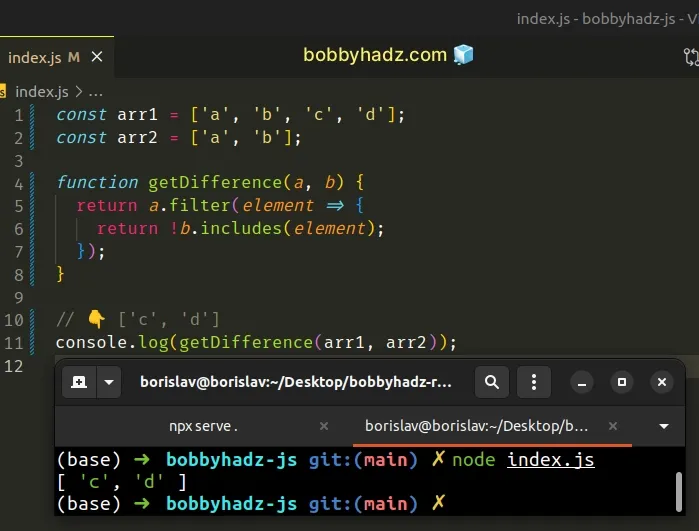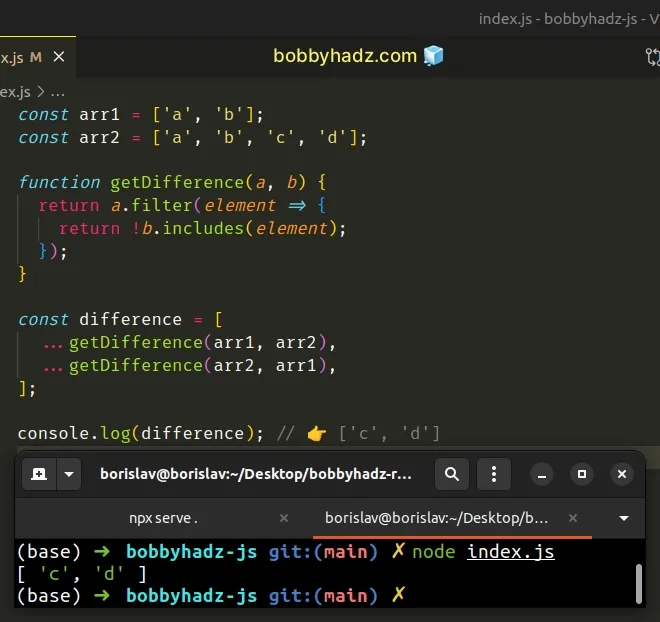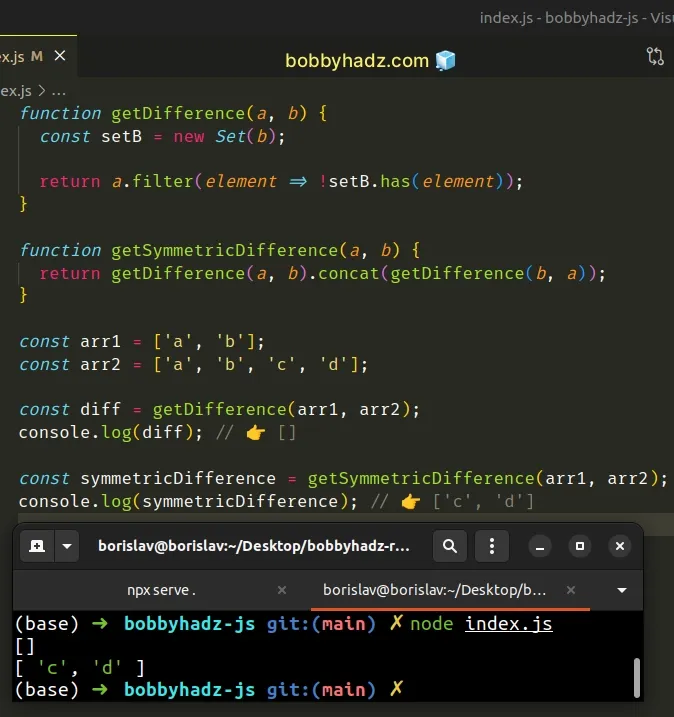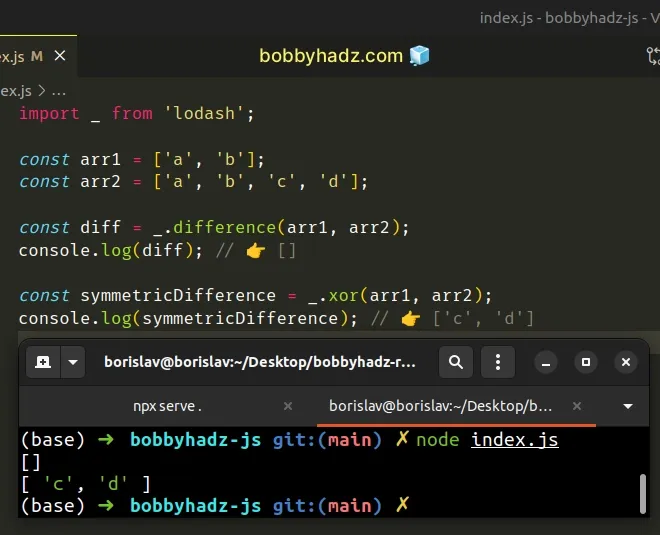# Get the Difference between Two Arrays in JavaScriptLast updated: Dec 27, 2022
4 min## #Get the Difference between two Arrays in JavaScript

To get the difference between two arrays:

1. Use the `filter()` method to iterate over the first array.
2. Check if each element is not contained in the second array.
3. Repeat the steps, but this time iterate over the second array.
index.js
```Copied!```const arr1 = ['a', 'b', 'c', 'd'];
const arr2 = ['a', 'b'];

function getDifference(a, b) {
return a.filter(element => {
return !b.includes(element);
});
}

// 👇️ ['c', 'd']
console.log(getDifference(arr1, arr2));
``````The function we passed to the Array.filter method gets invoked with each element in the array.

On each iteration, we check if the element is NOT contained in the other array.

The `filter` method returns an array containing the elements that meet the condition. In other words, the elements from the first array that are not contained in the second array.

However, this doesn't return the complete (symmetric) difference between the arrays, because we only check if the elements from the first array are not contained in the second array.
We didn't check if the elements from the second array are not contained in the first array.
index.js
```Copied!```const arr1 = ['a', 'b'];
const arr2 = ['a', 'b', 'c', 'd'];

function getDifference(a, b) {
return a.filter(element => {
return !b.includes(element);
});
}

// 👇️ []
console.log(getDifference(arr1, arr2));
``````

We switched the values of the `arr1` and `arr2` variables. Now, `arr2` contains 4 elements.

Since we only iterate over `arr1`, which only has `2` elements that are both contained in `arr2`, the method returns an empty array.

We would have expected a return value of `['c', 'd']` instead.

## #Getting the complete difference between two arrays

We need to call the `getDifference()` method two times and combine the results to resolve the issue.

index.js
```Copied!```const arr1 = ['a', 'b'];
const arr2 = ['a', 'b', 'c', 'd'];

function getDifference(a, b) {
return a.filter(element => {
return !b.includes(element);
});
}

const difference = [
...getDifference(arr1, arr2),
...getDifference(arr2, arr1)
];

console.log(difference); // 👉️ ['c', 'd']
``````The code sample returns the symmetric difference between the two arrays.

Here's what we did to get this working:

1. Call the `filter` method on the first array and return only the elements that are not contained in the second array.
2. Call the `filter` method on the second array and return only the elements that are not contained in the first array.
3. We combined the results from the two arrays into a third array using the spread syntax (...).

An easy way to think about the spread syntax (...) is that we are unpacking the values of one array into another array.

Now our example is complete and returns the symmetric difference between the two arrays.

You can also use the `concat()` method to join the results.

index.js
```Copied!```function getDifference(a, b) {
return a
.filter(element => !b.includes(element))
.concat(b.filter(element => !a.includes(element)));
}

const arr1 = ['a', 'b'];
const arr2 = ['a', 'b', 'c', 'd'];

console.log(getDifference(arr1, arr2)); // 👉️ ['c', 'd']
``````

The `getDifference()` function takes two arrays and returns the symmetric difference between the two arrays.

We used the `filter()` method to get the elements in the first array that are not contained in the second array.

We used the `filter()` method again to get the elements in the second array that are not contained in the first array.

The last step is to use the `Array.concat()` method to combine the two arrays we got from the `filter()` method calls.

You can also use a `Set` object to get the difference between two arrays.

## #Get the difference between two arrays using a `Set` object

This is a three-step process:

1. Convert the second array to a `Set` object.
2. Use the `filter()` method to iterate over the first array.
3. Check if each element of the array is not contained in the `Set`.
index.js
```Copied!```function getDifference(a, b) {
const setB = new Set(b);

return a.filter(element => !setB.has(element));
}

function getSymmetricDifference(a, b) {
return getDifference(a, b).concat(getDifference(b, a));
}

const arr1 = ['a', 'b'];
const arr2 = ['a', 'b', 'c', 'd'];

const diff = getDifference(arr1, arr2);
console.log(diff); // 👉️ []

const symmetricDifference = getSymmetricDifference(arr1, arr2);
console.log(symmetricDifference); // 👉️ ['c', 'd']
``````We used the `Set()` constructor to convert the second array to a `Set` in the `getDifference()` function.

On each iteration, we use the `Set.has()` method to check if the current array element is not contained in the `Set`.

If you need to get the symmetric difference between the two arrays, use the `getSymmetricDifference()` function instead.

The function calls the `getDifference()` function twice and concatenates the results.

You can also use `lodash` to get the difference between two arrays.

## #Get the Difference between two Arrays using Lodash

Alternatively, you can use the `lodash` library:

1. Install the `lodash` library.
shell
```Copied!```npm install lodash

# 👇️ only if you use TypeScript
npm install @types/lodash --save-dev
``````
1. Use the `difference()` method to get the difference between the arrays.
2. Use the `xor()` method to get the symmetric difference between the arrays.
index.js
```Copied!```import _ from 'lodash';

const arr1 = ['a', 'b'];
const arr2 = ['a', 'b', 'c', 'd'];

const diff = _.difference(arr1, arr2);
console.log(diff); // 👉️ []

const symmetricDifference = _.xor(arr1, arr2);
console.log(symmetricDifference); // 👉️ ['c', 'd']
``````The difference method returns the difference between two or more arrays.

If you need to get the symmetric difference between the arrays, use the xor method.

I've also written an article on how to get the difference between two arrays of objects in JS.